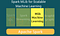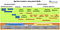# Spark for Machine Learning using Python and MLlibhttps://www.dezyre.com/article/spark-mllib-for-scalable-machine-learning-with-spark/339

MLlib is Spark’s machine learning (ML) library. Its goal is to make practical machine learning scalable and easy. At a high level, it provides tools such as:

• ML Algorithms: common learning algorithms such as classification, regression, clustering, and collaborative filtering

# Prerequisites :

Before we begin, please set up the Python and Apache Spark environment on your machine. Head over to this blog here to install if you have not done so.

We will also be using MLlib module from Python in our virtual environment later which is built in by default with Spark .

# Spark MLlib :

Apache Spark offers a Machine Learning API called MLlib. PySpark has this machine learning API in Python as well. It supports different kind of algorithms, which are mentioned below :https://sites.google.com/site/irisforbigdata/3-supporting-tool1/machine-learning-lib-spark
• mllib.classification − The spark.mllib package supports various methods for binary classification, multiclass classification and regression analysis. Some of the most popular algorithms in classification are Random Forest, Naive Bayes, Decision Tree, etc.

# Benefits of Spark MLlib :

• Spark MLlib is tightly integrated on top of Spark which eases the development of efficient large-scale machine learning algorithms as are usually iterative in nature.

# Basic Statistics and Exploratory Data Analysis

## Summary statistics :

We get the column summary statistics for `RDD[Vector]` through the function `colStats` available in `Statistics`.

`colStats()` returns an instance of `MultivariateStatisticalSummary`, which contains the column-wise max, min, mean, variance, and number of nonzeros, as well as the total count.

## Correlations :

Calculating the correlation between two series of data is a common operation in Statistics. In MLlib we provide the flexibility to calculate pairwise correlations among many series. The supported correlation methods are currently Pearson’s and Spearman’s correlation.

`Statistics` provides methods to calculate correlations between series. Depending on the type of input, two `RDD[Double]`s or an `RDD[Vector]`, the output will be a `Double` or the correlation `Matrix` respectively.

## Stratified sampling :

Unlike the other statistics functions, which reside in MLLib, stratified sampling methods, `sampleByKey` and `sampleByKeyExact`, can be performed on RDD’s of key-value pairs. For stratified sampling, the keys can be thought of as a label and the value as a specific attribute.

## Random data generation :

Random data generation is useful for randomized algorithms, prototyping, and performance testing. MLlib supports generating random RDDs with i.i.d. values drawn from a given distribution: uniform, standard normal, or Poisson.

`RandomRDDs` provides factory methods to generate random double RDDs or vector RDDs. The following example generates a random double RDD, whose values follows the standard normal distribution `N(0, 1)`, and then map it to `N(1, 4)`.

# Feature extraction and transformation :

## TF-IDF :

we start with a set of sentences. We split each sentence into words using `Tokenizer`. For each sentence (bag of words), we use `HashingTF` to hash the sentence into a feature vector. We use `IDF` to rescale the feature vectors; this generally improves performance when using text as features. Our feature vectors could then be passed to a learning algorithm.

Refer to the HashingTF Python docs and the IDF Python docs for more details on the API.

## Word2Vec :

We start with a set of documents, each of which is represented as a sequence of words. For each document, we transform it into a feature vector. This feature vector could then be passed to a learning algorithm.

Refer to the Word2Vec Python docs for more details on the API.

## Tokenizer :

Tokenization is the process of taking text (such as a sentence) and breaking it into individual terms (usually words). A simple Tokenizer class provides this functionality. The example below shows how to split sentences into sequences of words.

Refer to the Tokenizer Python docs and the RegexTokenizer Python docs for more details on the API.

## N-gram :

N-gram is a sequence of nn tokens (typically words) for some integer nn. The `NGram` class can be used to transform input features into nn-grams.

Refer to the NGram Python docs for more details on the API.

# Classification and Regression :

## Binary classification (SVMs, logistic regression) :

The following example shows how to load a sample dataset, build Logistic Regression model, and make predictions with the resulting model to compute the training error.

The following example demonstrate how to load training data, parse it as an RDD of LabeledPoint. The example then uses LinearRegressionWithSGD to build a simple linear model to predict label values. We compute the mean squared error at the end to evaluate goodness of fit.

## Decision Trees :

Classification :

The example below demonstrates how to load a LIBSVM data file, parse it as an RDD of `LabeledPoint` and then perform classification using a decision tree with Gini impurity as an impurity measure and a maximum tree depth of 5. The training error is calculated to measure the algorithm accuracy.

Regression :

The example below demonstrates how to load a LIBSVM data file, parse it as an RDD of `LabeledPoint` and then perform regression using a decision tree with variance as an impurity measure and a maximum tree depth of 5. The Mean Squared Error (MSE) is computed at the end to evaluate goodness of fit.

## Naive Bayes :

MLlib supports multinomial naive Bayes, which is typically used for document classification.

NaiveBayes implements multinomial naive Bayes. It takes an RDD of LabeledPoint and an optional smoothing parameter `lambda` as input, and output a NaiveBayesModel, which can be used for evaluation and prediction.

## Clustering :

In the following example after loading and parsing data, we use the KMeans object to cluster the data into two clusters. The number of desired clusters is passed to the algorithm. We then compute Within Set Sum of Squared Error (WSSSE). You can reduce this error measure by increasing k.

In fact the optimal k is usually one where there is an “elbow” in the WSSSE graph.

# Ressources/References :

Committed lifelong learner. I am passionate about machine learning, data engineering and currently working as a datascientist.

## More from Jaafar Benabderrazak

Committed lifelong learner. I am passionate about machine learning, data engineering and currently working as a datascientist.